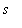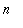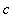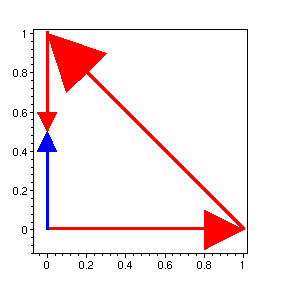Function: arrow2d - plot an arrow as a line with a triangular head.

Calling sequence:

arrow2d(base,vect);
Parameters:

base - base of the arrow, a 2D point ( list or vector)

vect - displacement (tip - base), a 2D point (list or vector)

Optional arguments:

scalefactor=to multiply length by real constant.

thickness=for thickness of the arrow shaft (should be 0, 1, 2 or 3, default is 3).

colour=to specify arrow colour (default is red).

other 2D plot options

Description:

This function creates a 2D plot data object which, when displayed, is an arrow from base to base+vect (or base+s*vect if the option scalefactor=s is specified).

For an arrow from base to tip , use arrow2d(base,tip-base) .

Unless the option scaling=constrained ("Projection, Constrained" on the plot menu or "1-1" on the context bar) is used, the shape of the arrowhead may be distorted.

While it is mainly provided for use by the curvarrows function, this function can be used on its own. Its result would usually be combined with other plot structures by the function display from the plots package.

This function is part of the package surfarro in the Maple Advisor Database library, and must be loaded before use by the command with(surfarro); or with(surfarro,arrow2d); .

Examples:

> with(surfarro,arrow2d):

> a1:= arrow2d([0,0],[1,0]):
a2:= arrow2d([1,0],[0,1]-[1,0]):
a3:= arrow2d([0,1],[0,-1],scalefactor=1/2):
a4:= arrow2d([0,0],[0,1/2],colour=blue):
plots[display]({a1,a2,a3,a4},scaling=constrained,axes=box);